# HBSE 10th Class Maths Important Questions Chapter 5 Arithmetic Progressions

Haryana State Board HBSE 10th Class Maths Important Questions Chapter 5 Arithmetic Progressions Important Questions and Answers.

## Haryana Board 10th Class Maths Important Questions Chapter 5 Arithmetic Progressions

Question 1.
For what value of k will k + 9, 2k – 1 and 2k + 7 are the consecutive terms of an AP?
Solution :
The consecutive terms of an AP are k + 9, 2k – 1 and 2k + 7, then
2k – 1 = $$\frac{k+9+2 k+7}{2}$$
⇒ 4k – 2 = 3k + 16
⇒ 4k – 3k = 16 + 2
⇒ k = 18.Question 2.
Find how many integers between 200 and 500 are divisible by 8.
Solution :
The numbers divisible by 8 between 200 and 500 are : 208, 216, 224,…..496, which an AP.
Here, a = 208, d = 8 and an = l = 496
We know that an = a + (n – 1)d
⇒ 496 = 208 + (n – 1) × 8
⇒ 496 – 208 = 8n – 8
⇒ 288 = 8n – 8
⇒ 288 + 8 = 8n
⇒ $$\frac {296}{8}$$ = n
⇒ n = 37
Hence, required integer divisible by 8 = 37.

Question 3.
Find the middle term of an AP: 213, 205, 197……..
Solution :
The given sequence of an AP is 213, 205, 197, ………37
Here, a = 213, d = 205 – 213 = -8, l = 37
Let the number of terms ben
an = l = a + (n – 1)d
⇒ 37 = 213 + (n – 1) × (- 8)
⇒ 37 = 213 – 8n + 8
⇒ 8n = 221 – 37
⇒ n = $$\frac {184}{8}$$ = 23
The middle term will be $$\frac{23+1}{2}$$ i.e 12th
So, a12 = 213 + (12 – 1) × (-8)
= 213 – 88 = 125
Hence, middle term os AP is 125.Question 4.
For what value of n are the nth terms of two APs : 63, 65, 67 …..and 3, 10, 17……..
Solution :
The two APs are 63, 65, 67,…. and 3, 10, 17……
Let a, d and A, D be Ist term and common difference of two given APs
Here, a = 63, d = 65 – 63 = 2 And
A = 3, D = 10 – 3 = 7
nth terms of two APs are eqal
an = An
⇒ a + (n – 1)d = A + (n – 1)D
⇒ 63 + (n – 1) × 2 = 3 + (n – 1) × 7
⇒ 63 + 2n – 2 = 3 + 7n – 7
⇒ 2n + 61 = 7n – 4
⇒ 61 + 4 = 7n – 2n
⇒ 65 = 5n
⇒ n = $$\frac {65}{5}$$ = 13
Hence, 13th term of two given APs are equal.

Question 5.
Write the nth terms of the AP:
$$\frac{1}{m}, \frac{1+m}{m}, \frac{1+2 m}{m}$$ (CBSE-Comptt 2017)
Solution :
The given sequence of an APs is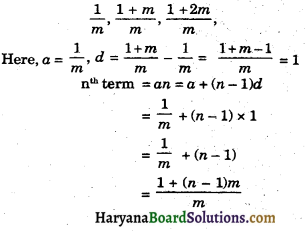Question 6.
Which term of the AP. 20, 19$$\frac {1}{4}$$, 18$$\frac {1}{2}$$, 17$$\frac {3}{4}$$, is the first negative term. (CBSE 2020)
Solution :
The given sequence of AP is
20, 19$$\frac {1}{4}$$, 18$$\frac {1}{2}$$, 17$$\frac {3}{4}$$, …………
Here, a = 20, d = 19$$\frac {1}{4}$$ – 20 = $$\frac {-3}{4}$$
Let nth term of an AP be the first negative term, then,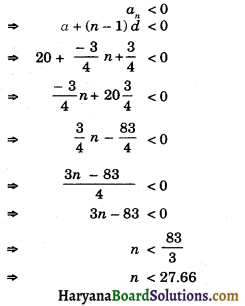Hence, 28th term of the AP is the first negative term.

Question 7.
If m times the mth term of an AP is equal to n times its nth term and m ≠ n, show the (m + n)th term of AP is zero. [CBSE 2019]
Solution :
Let a be first term and d be common difference of AP.
We have, mam = nan
⇒ m[a + (m – 1)d] = n[a + (n – 1)d]
⇒ ma + m2d – md = na + n2d – nd
⇒ m2d – n2d – md + nd = na – ma
⇒ d(m2 – n2) – d(m – n) = a(n – m)
⇒ d[m2 – n2 – (m – n)] = a(n – m)
⇒ d[(m + n) (m – n) – (m – n)] = – a(m – n)
⇒ d[(m – n) (m + n – 1)] = – a(m – n)
⇒ d(m + n – 1) = $$\frac{-a(m-n)}{(m-n)}$$
⇒ d(m + n – 1) = – a
⇒ d = – $$\frac{a}{m+n-1}$$
Now, a(m+n) = a + (m + n – 1)d
⇒ a(m+n) = a + (m + n – 1) × – $$\frac{a}{(m+n-1)}$$
⇒ a(m+n) = a – a = 0
Hence, (m + n)th term = 0.Question 8.
A manufactures of TV set produce 720 sets in fourth year and 1080 set in the sixth year, Assuming that the production increase uniformly by a fixed number every year, then find total production in first 9 year.
Solution :
Production of TV sets in 4th year = 720,
Production of TV sets in 6th year = 1080
Let a and d be first term and common difference respectively then,
a4 = a + (4 – 1)d
⇒ 720 = a + 3d
⇒ a + 3d = 720 …….(1)
And a6 = a + (6 – 1)d
⇒ 1080 = a + 5d
⇒ a + 5d = 1080 …….(2)
Substracting equ. (2) from equ. (1), We get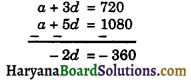⇒ d = $$\frac {-360}{-2}$$ = 180
Substituting the value of d in equ. (1), Weget
a + 3 × 180 = 720
⇒ a + 540 = 720
⇒ a = 720 – 540
⇒ a = 180
Production of TV sets increases uniformly by a fixed numbers.
So, Total productions of TV sets in first 9 year = a9
a9 = a + (9 – 1) 180
= 180 + 8 × 180
= 180 + 1440 = 1620
Hence, production in first 9th year = 1620.

Question 9.
Find the sum of the first 20 terms of the following AP : 1, 4, 7, 10,……..
Solution :
The given sequence of AP is 1, 4, 7, 10 …………
Here, a = 1, d = 4 – 1 = 3, n = 20
We know that
Sn = $$\frac {n}{2}$$[2a + (n – 1)d]
= $$\frac {20}{2}$$(2 × 1 + (20 – 1) × 3]
= 10 (2 + 57)
= 10 × 59 = 590
Hence, sum of first 20 term = 590Question 10.
Find the sum of all two digit natural numbers which are divisible by 4.
Solution :
The all two digit numbers which are divisible by 4 are :
12, 16, 20,…………..96
Here, a = 12, an = l =96, d = 16 – 12 = 4
∴ an = a + (n – 1)d
⇒ 96 = a + (n – 1)d
⇒ 96 = 12 + (n – 1) × 4
⇒ 96 = 12 + 4n – 4
⇒ 96 – 8 = 4n
⇒ 88 = 4n
⇒ n = $$\frac {88}{4}$$ = 22
Sum of first 22 terms = S22
∴ S22 = $$\frac {22}{2}$$[12 + 96]
= 11 × 108 = 1188
Hence, sum of all two digit numbers divisible by 4 = 1188

Question 11.
How many terms of AP – 6, $$\frac {-11}{2}$$, – 5, $$\frac {-9}{2}$$, ………. are needed to give their sum zero.
Solution :
The given sequence of AP is
– 6, $$\frac {-11}{2}$$, – 5, $$\frac {-9}{2}$$, ……….
Here, a = – 6, d = $$\frac {-11}{2}$$ – (-6) = $$\frac{-11}{2}+\frac{12}{2}=\frac{1}{2}$$
and Sn = 0 (given)
Let AP has n terms
Sn = 0
⇒ $$\frac {n}{2}$$[2a + (n – 1)d] = 0
⇒ $$\frac {n}{2}$$[2 × (-6) + (n – 1) × $$\frac {1}{2}$$] = 0
⇒ $$\frac {n}{2}$$[- 12 + $$\frac{n}{2}-\frac{1}{2}$$] = 0
⇒ $$\frac {n}{4}$$[- 24 + n – 1] = 0
⇒ n[- 25 + n] = 0
⇒ n2 – 25n = 0
⇒ n(n – 25) = 0
⇒ n = 0 or n – 25 = 0
⇒ n = 0 or = n = 25
Reject n = 0
Hence, terms needed = 25Question 12.
How many terms of the AP : 45, 39, 33………must be taken so that their sum is 180 ? explain the double answer.
Solution :
The given sequence of AP is 45, 39, 33, ………….
Here, a = 45, d = 39 – 45 = – 6 and Sn = 180 (given)
Let AP has n terms
Sn = 180
⇒ $$\frac {n}{2}$$[2a + (n – 1)d] = 180
⇒ $$\frac {n}{2}$$[2 × 45 + (n – 1)(-6)] = 180
⇒ $$\frac {n}{2}$$[90 – 6n + 6] = 180
⇒ $$\frac {n}{2}$$[96 – 6n] = 180
⇒ 96n – 6n2 = 360
⇒ 6n2 – 96n + 360 = 0
⇒ n2 -16n + 60 = 0
⇒ n2 – (10 + 6)n + 60 = 0
⇒ n – 10n – 6n + 60 = 0
⇒ n(n – 10) – 6 (n – 10) = 0
⇒ (n – 10)(n – 6) = 0
⇒ n = 10, or n = 6
Hence, 10 or 6 terms must be taken

Question 13.
Solve the equation : 1 + 4 + 7 + 10 + …… +x = 287
Solution :
The given equation is 1 + 4 + 7 + 10 +……+ x = 287
Here, a = 1, d = 4 – 1 = 3, an = x and Sn = 287
∴ an = a + (n – 1)d
⇒ x = 1 + (n – 1) × 3
⇒ x = 1 + 3n – 3
⇒ x = 3n – 2 ………….(i)
Also Sn = $$\frac {n}{2}$$[1 + l]
⇒ 287 = $$\frac {n}{2}$$[1 + x]
⇒ 287 = $$\frac {n}{2}$$[1 + 3n – 2]
⇒ 287 = $$\frac {n}{2}$$[3n – 1]
⇒ 574 = 3n2 – n
⇒ 3n2 – n – 574 = 0
n = $$\frac{1 \pm \sqrt{(-1)^2-4 \times 3 \times(-574)}}{2 \times 3}$$
= $$\frac{1 \pm \sqrt{1+6888}}{6}=\frac{1 \pm \sqrt{6889}}{6}=\frac{1 \pm 83}{6}$$
⇒ n = $$\frac{1+83}{6}$$ or n = $$\frac{1-83}{6}$$
⇒ n = 14 or n = $$\frac {- 41}{3}$$ (Reject)
So, putting the value of n in equation (1), we get
x = 3 × 14 – 2 = 42 – 2 = 40
Hence, x = 40Question 14.
If second and third terms of an AP are 3 and 5 respectvely, then find the sum of first 20 terms of it.
Solution :
Let a be first term and common difference bed of an AP
a2 = 3
⇒ a + d = 3 ………..(1)
And a3 = 5
⇒ a + 2d = 5 ……….(2)
Subtracting equ. (2) from equ. (1), we get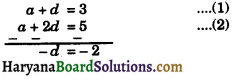⇒ d = 2
Substituting the value of d in equ (1), we get
a + 2 = 3
⇒ a = 1
S20 = $$\frac {20}{2}$$[2 × 1 + (20 – 1) × 2]
= 10 [2 + 38]
= 10 × 40 = 400
Hence,
S20 = 400

Question 15.
If the sum of the first n terms of an AP is $$\frac {1}{2}$$(3n2 + 7n), then find its nth terma. Hence write its 20th terms.
Solution :
We have
Sn = $$\frac {1}{2}$$[3n2 + 7n]
S1 = $$\frac {1}{2}$$[3 × 12 + 7 × 1]
= $$\frac {1}{2}$$ × 10 = 5
S2 = $$\frac {1}{2}$$[3 × 22 + 7 × 2]
= $$\frac {1}{2}$$[12 + 14]
= $$\frac {1}{2}$$ × 26 = 13
∴ a1 = S1 = 5, a2 = S2 – S1 = 13 – 5 = 8
d = 8 – 5 = 3
So, AP is 5, 8, 11, ……………….
Nth term (an) =a + (n – 1)d
= 5 + (n – 1) × 3
= 5 + 3n – 3
= 3n + 2
And a20 = 5 + (20 – 1) × 3
= 5 + 57 = 62.
Hence, AP is, 5, 8, 11, ………… and a20 = 62Question 16.
If the sum of first four terms of an AP is 40 and that of first 14 terms is 280. Find the sum of its n terms.
Solution :
Let first terms be a and common difference be d
S4 = 40 (given)
⇒ $$\frac {4}{2}$$[2a + (4 – 1) × d] = 40
⇒ 2[2a + 3d] = 40
⇒ 2a + 3d = 20 …. (1)
And S14 = 280
⇒ $$\frac {14}{2}$$[2a + (14 – 1)d] = 280
⇒ 2a + 13d = $$\frac {280}{7}$$
⇒ 2a + 13d = 40 ………(2)
Subtracting the equ (2) from equ. (1), we get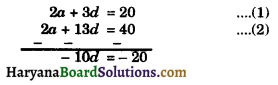⇒ d = $$\frac {- 20}{- 10}$$ = 2
Substituting the value of d in the equ. (1), we get
2a + 3 × 2 = 20
⇒ 2a + 6 = 20
⇒ 2a = 20 – 6 = 14
⇒ a = $$\frac {14}{2}$$ = 7
Its sum of nth term (Sn) = $$\frac {n}{2}$$[2a + (n – 1)d]
= $$\frac {n}{2}$$[2 × 7 + (n – 1) × 2]
= $$\frac {n}{2}$$[14 + 2n – 2]
= $$\frac {n}{2}$$[12 + 2n]
= n (6 + n) = n2 + 6n
Hence, sum of its nth = n2 + 6n.

Question 17.
Show that the sum of an AP whose first term is a, the second term b and the last term c, is equal to $$\frac{(a+c)(b+c-2 a)}{2(b-a)}$$.
Solution :
We have, first term = a
second term = b
Then, common difference (d)
= b – a
last term (l) = c
Let n be the number of terms in the AP
∴ an = l = c
⇒ a + (n – 1) × (b – a) = c
⇒ (n – 1) (b – a) = c – a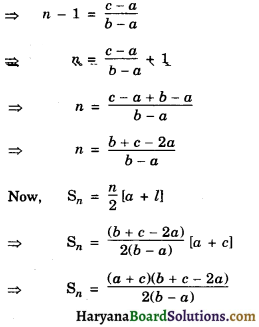Hence Proved.

Question 18.
Find the sum of all 11 terms of an A.P. whose middle terms is 30.
Solution :
Let first term be a and common difference be d of, AP consisting of 11 terms, ($$\frac{11+1}{2}$$)th i.e. 6th terms is middle terms. But it is given that middle most term is 30.
∴ a6 = 30
⇒ a + (6 – 1)d = 30
⇒ a + 5d = 30
Sum of 11 terms (S11) = $$\frac {11}{2}$$[2a + (11 – 1)d]
= $$\frac {11}{2}$$[2a + 10d]
= 11 (a + 5d)
= 11 × 30 [using eq. (1)]
= 330
Hence, S11 = 330.Question 19.
If in an A.P. the sum of first m times is n and the sum of its first n terms is m, then prove that the sum of its first (m + n) terms is -( m + n)
Solution :
Let first term be a and common difference be d of given A.P. then.
Sm = n (given)
⇒ $$\frac {m}{2}$$[2a + (m – 1)d] = n
⇒ 2ma + m (m – 1)d = 2n ………….(1)
And Sn = m (given)
⇒ $$\frac {n}{2}$$[2a + (n – 1)d] = m
⇒ 2na + n(n – 1)d = 2m ………….(2)
Substracting eq. (2) from eq. (1), we get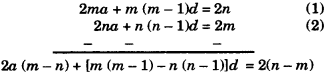2a(m – n) + [m (m – 1) – n(n – 1)]d = 2(n – m)
⇒ 2a(m – n) + [(m2 – m – n2 + n)]d = – 2(m – n)
⇒ 2a(m – n) + [(m2 – n2) – (m – n)]d = – 2(m – n)
⇒ 2a(m – n) + [(m + n) (m – n) – (m – n)d)] = 2(m – n)
(m – n)[2a + (m + n – 1)d] = – 2(m – n)
⇒ 2a + (m + n – 1)d = $$\frac{-2(m-n)}{(m-n)}$$
2a + (m + n – 1)d = – 2 ……..(3)
Now, S (m+n) = $$\frac{(m+n)}{2}$$[2a + (m + n – 1)d]
= $$\frac{(m+n)}{2}$$ × (-2)
(using equation) ….(3)
= – (m + n)
Hence, S (m + n) = – (m + n)
Hence proved.

Question 19.
If mth term of AP is $$\frac {1}{n}$$ and nth term is , find the sum of first mn terms.
Solution :
Let the first term of an AP is a and its common difference be d. them.
am = $$\frac {1}{n}$$ …………(1)
⇒ a + (m – 1)d = $$\frac {1}{n}$$
And an = $$\frac {1}{m}$$
⇒ a + (n – 1)d = $$\frac {1}{m}$$ …………(2)
Subtracting the equation (2) from (1), We get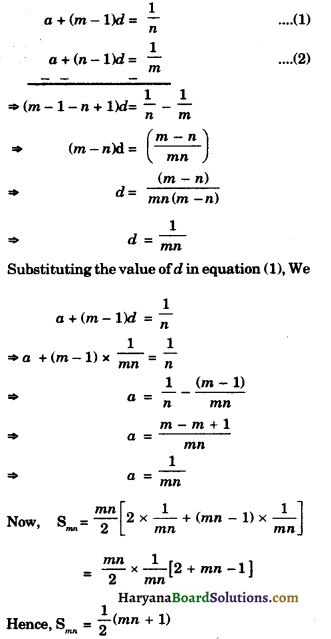Question 20.
A Motor car travels 175 km distance from place A to B, at a uniform speed 70km/ hr, passes through all ten green traffic signals. Due to heavy traffic it stops for one minute at first signal 3 minutes at second signal, 5 minutes at third signal and so on stops for 19 minutes at tenth signal How much total time to reach at the place B. Solve by suitable mathematical method.
Solution :
Distance travelled by car (s) = 175km.
Speed of a car (s) = 70km/hr.
Time taken by a car = $$\frac {D}{S}$$
to reach place AtoB = $$\frac {175}{70}$$ hrs = $$\frac {5}{2}$$hours.
Due to heavy traffic a car stops at signal Iat to signal s 10 Time taken by a car at signal Ist to signal 10 = 1 min + 3 min + 5 min + ——- 19
Here a1 = – 1, a2 = – 3, a3 = 5 and am = 19, n = 10.
Since, a2 – a1 = 3 – 1 = 2, a3 – a2 = 5 – 3 = 2,
a3 – a2 = a2 – a1
So, the given sequence form an AP
∴ S10 = $$\frac {n}{2}$$[a + l]
= $$\frac {10}{2}$$[1 + 19]
= 5 × 20 = 100 min.
So, car stops at ten signal = 100 min
= $$\frac {100}{60}$$hrs
= 1$$\frac {40}{60}$$hrs
= 1$$\frac {2}{3}$$ = $$\frac {5}{3}$$hrs
Total time taken by car = $$\frac{5}{2}+\frac{5}{3}=\frac{15+10}{6}$$
= $$\frac {25}{6}$$ = 4$$\frac {1}{6}$$hrsQuestion 21.
A Car travels 260km distance from place A to place B, at a uniform speed 65 km/hr passes through all thirreen green traffic signals, 4 minutes at first signal, 7 minutes at second signal 10 minutes at third signal and soon stops for 40 minutes at thirteen signal. How much total it takes to reach at the place B? Solve by suitable mathematical method.
Solution :
Distance covered from place A to B = 260 km/hr.
Speed of a car = 65km/hr
Time taken by a car = $$\frac{260}{65}=\frac{20}{5}$$ = 4 hrs.
Car stops at thirteen green signals So, time taken by car at 13 green signals in
min = 4 + 7 + 10 + …….. 40
Since, a2 – a1 = 7 – 4 = 3, a3 – a2 = 10 – 7 = 3
a3 – a2 = a2 – a1 =
So, the given sequence form an AP
Here, a = 4, d = 3, an = 40 and n = 13
∴ S13 = $$\frac {n}{2}$$[a + l]
= $$\frac {13}{2}$$[4 + 40]
= $$\frac {13}{2}$$ × 44
= 13 × 12 = 156 min.
So, time taken (extra) by a car = 156 min
= $$\frac {156}{60}$$ hrs
2$$\frac {36}{60}$$hrs = 2$$\frac {3}{5}$$hrs = $$\frac {13}{5}$$hrs
Total time taken by a car
= 4 + 2$$\frac {3}{5}$$hrs
= 6$$\frac {2}{5}$$hrs

Fill in the Blanks

Question 1.
If a, b, c are in AP, then ………… = $$\frac{a+c}{2}$$ and b is called the airthmetic mean.
Solution :
bQuestion 2.
The constant difference between any two consecutive terms of an AP is called the ……………. of the AP.
Solution :
commondifference

Question 3.
= $$\frac {x}{2}$$[2a + (n – 1)d]
Solution :
Sn

Question 4.
The common differeence of an AP can be positive, negative or ………..
Solution :
zero

Question 5.
An arithmetic progression is a list of numbers in which each term is obtained by adding or substracting a fixed number of the …………. term expect the first term.
Solution :
precedingQuestion 6.
A ……….. may be difined as an arrangement of numbers in some definite order and according to some rule.
Solution :
sequence.

Multiple Choice Questions

Question 1.
The common difference of the AP.
$$\frac{1}{p}, \frac{1-p}{p}, \frac{1-2 p}{p}$$ is :
(a) 1
(b) $$\frac {1}{p}$$
(c) – 1
(d) – $$\frac {1}{p}$$
Solution :
(c) The sequence of given AP is
$$\frac{1}{p}, \frac{1-p}{p}, \frac{1-2 p}{p}$$, ………..
Common difference (d)
=$$\frac{1-\mathrm{P}}{\mathrm{P}}-\frac{1}{P}=\frac{1-\mathrm{P}-1}{\mathrm{P}}=\frac{-\mathrm{P}}{\mathrm{P}}$$ = – 1

Question 2.
The nth term of the AP. a, 3a, 5a, ……….
(a) na
(b) (2n – 1)a
(c) 2(2n + 1)
(d) 2na
Solution :
(b) The sequence of given AP is a, 3a, 5a …….
Here,
a1 = a, d = 3a – a = 2a
nth term (an) = a + (n – 1)d .
= a + (n – 1) × 2a
= a + 2na – 2a
= 2na – a
= a (2n – 1)Question 3.
Value of x for which 2x, (x + 10) and (3x + 2) are there consecutive terms of an AP are :
(a) 6
(b) – 6
(c) 18
(d) – 18
Solution :
(a) Three consecutive terms of AP are 2x, (x + 10) and (3x + 2) Then,
x + 10 = $$\frac{2 x+3 x+2}{2}$$
⇒ 2x + 20 = 5x + 2
⇒ 20 – 2 = 5x – 2x
⇒ 18 = 3x
⇒ x = $$\frac {18}{3}$$ = 6

Question 4.
The first term of AP is p and common difference is q, then its 10th term is :
(a) q + qp
(b) p – 9q
(c) p + 9q
(d) 2p + 9q.
Solution :
(c) p + 9q

a = p, d = q
an = a + (n – 1)d
⇒ a10 = p + (10 – 1) = p + 9q

Question 5.
The 5th terms of the sequence defined by t1 = 2, t2 = 3 and tn = tn-1 + tn-2 for n ≥ 3 :
(a) 16
(b) 13
(c) 15
(d) 2.
Solution :
(b) t1 = 2, t2 = 3

and tn = tn-1 + tn-2
t3 = t2 + t1 = 3 + 2 = 5
t4 = t3 + t2 = 5 + 3 = 8
t5 = t4 + t3 = 8 + 5 = 13
Hence correct choice is (b).Question 6.
The sum of first 20 odd natural numbers is : ……………..
(a) 400
(b) 200
(c) 500
(d) 800.
Solution :
(a) Odd number is 1, 3, 5 ……
a = 1, d = 2, n = 20
Sn = $$\frac {n}{2}$$[2a + (n – 1)d]
= $$\frac {20}{2}$$[2 × 1 + 19 × 2]
= 10 × 40
= 400
So, correct choice is (a).